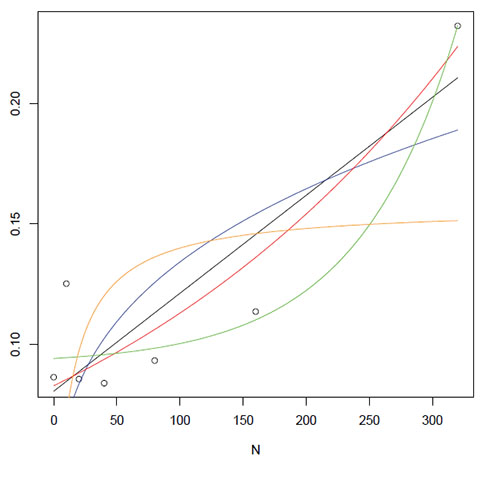Ecological Archives E096-084-A3

Lucía Vivanco, Irina C. Irvine, Jennifer B. H. Martiny. 2015. Nonlinear responses in salt marsh functioning to increased nitrogen addition. Ecology 96:936–947. http://dx.doi.org/10.1890/13-1983.1

Appendix C. Model fitting of plant, microbial, and sediment responses to nitrogen addition gradient, and a figure showing data fitting to five models and their Akaike values are presented.

Table C1. Results of fitting data to a linear, power, exponential, exponential with asymptote and Monod functions. The preferred model was selected based on the highest Akaike weight (in bold).

 Linear function Power function Exponential function Exponential with asymptopte function Monod function Plant characteristics Plant biomass_7 months (kg/m²) AICc 0.18 25.69 0.33 2.28 25.78 Akaike weight 0.44 0 0.41 0.15 0 Plant regrowth_14 months (kg/m²) AICc 11.62 17.42 13.47 7.19 18.2 Akaike weight 0.09 0.01 0.04 0.86 0 Leaf N content (%) AICc 9.9 31.68 10.26 1.32 31.7 Akaike weight 0.01 0 0.01 0.98 0 Microbial processes Methane flux (mg m-2 d-1) AICc 1.55 3.58 1.9 4.01 4.08 Akaike weight 0.36 0.13 0.3 0.11 0.1 Potential net N mineralization  (μg NH4-N·g-1 dry sediment·d-1) AICc -4.14 - 1.47 -3.79 -4.18 Akaike weight 0.34* - 0.02 0.29 0.35 Sediment characteristics Total N (kg/m²) TRE AICc -18.88 -8.87 -21.86 -23.95 -6.69 Akaike weight 0.06 0 0.25 0.7 0 Extractable ammonium  (μg NH4-N /g dry sediment) CSM AICc 59.04 59.64 65.59 60.74 59.36 Akaike weight 0.33 0.24 0.01 0.14 0.28 TRE AICc 42.42 47.82 33.64 32.94 - Akaike weight 0.01 0 0.41 0.58 -

*Data fit to linear and Monod functions similarly. Linear function was prefered due to parsimony.

Fig. C1. (below) Responses to the N addition gradient. The best fits of five functions to each dataset are shown: linear (y = ax + b) (black line), power (y = axb) (blue line), exponential with a horizontal asymptote at zero (y = aebx) (red line), a three-parameter exponential with a horizontal asymptote at c (y = aebx+ c) (green line), and Monod (y = a/(1 + bx-1) (orange line), where x is nitrogen concentration, and a, b, and c are constants. Each data point (circles) is an average ofn = 15, exceptfor panels (f) - (h) where n = 5 (see Methods).

(a) Plant biomass, total harvest after 7 months (kg/m²)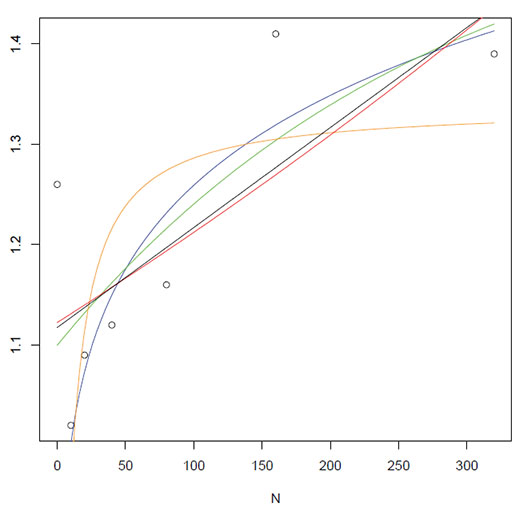(b) Plant biomass, regrowth after 14 months (kg/m²)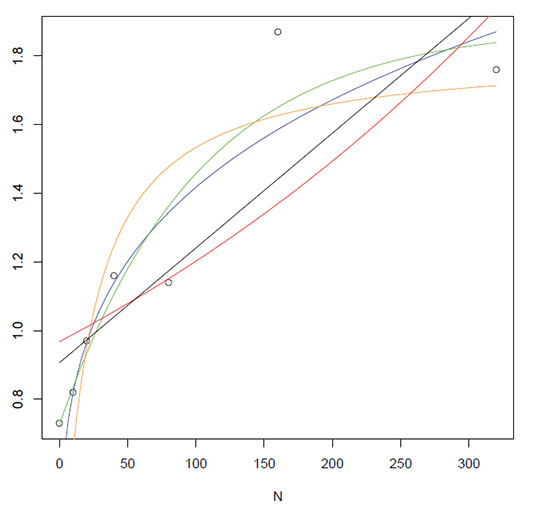(c) Leaf N content (%)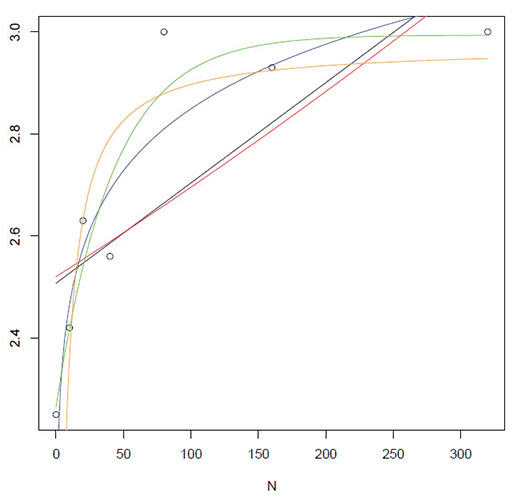(d) CH4 flux (mg CH4·m-2·d-1)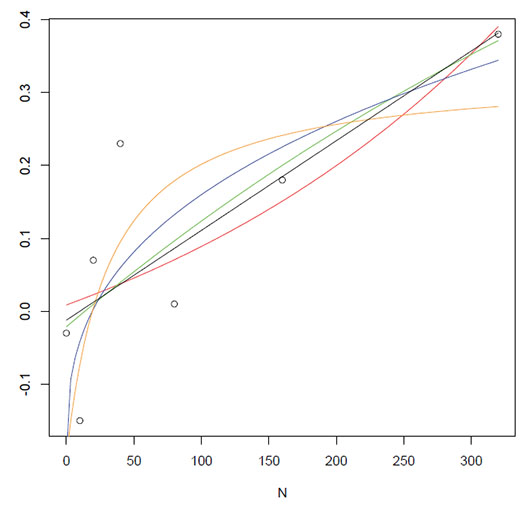(e) Potential net N mineralization (μg NH4-N g-1·dry sediment·d-1) (The power function could not be fit.)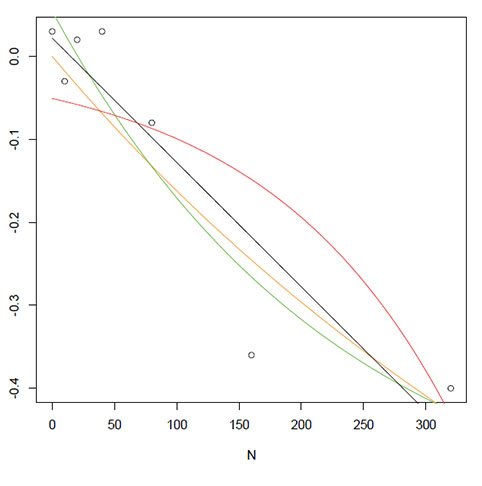(f) CSM Extractable NH4 (μg NH4-N /g dry sediment)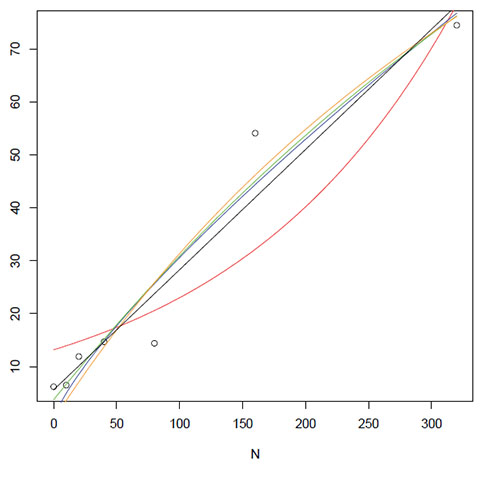(g) TRE Extractable NH4 (μg NH4-N /g dry sediment) (Note: The Monod function could not be fit.)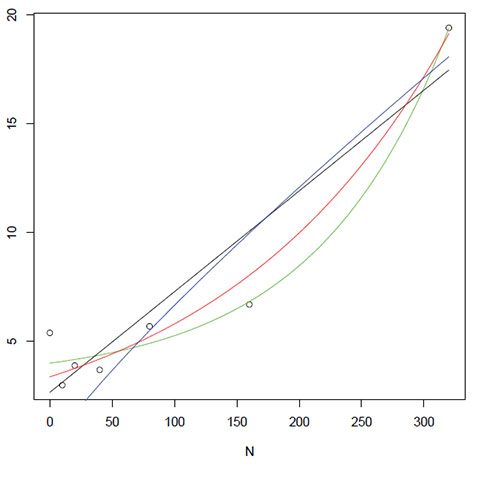(h) TRE Sediment N content (kg/m²)